Home | | Electronic Devices | Semiconductor Diode

# Semiconductor Diode

A semiconductor is a material which has electrical conductivity to a degree between that of a metal (such as copper) and that of an insulator (such as glass). Semiconductors are the foundation of modern electronics, including transistors, solar cells, light -emitting diodes (LEDs), quantum dots and digital and analog integrated circuits.

SEMICONDUCTOR DIODE

SEMICONDUCTOR

A semiconductor is a material which has electrical conductivity to a degree between that of a metal (such as copper) and that of an insulator (such as glass). Semiconductors are the foundation of modern electronics, including transistors, solar cells, light -emitting diodes (LEDs), quantum dots and digital and analog integrated circuits.

DIODE

Diode – Di + ode

Di means two and ode means electrode. So physical contact of two electrodes is known as diode and its important function is alternative current to direct current.

REVIEW OF INTRINSIC AND EXTRINSIC SEMICONDUCTORS

1 INTRINSIC SEMICONDUCTOR

An intrinsic semiconductor is one, which is pure enough that impurities do not appreciably affect its electrical behaviour. In this case, all carriers are created due to thermally or optically excited electrons from the full valence band into the empty conduction band. Thus equal numbers of electrons and holes are present in an intrinsic semiconductor. Electrons and holes flow in opposite directions in an electric field, though they contribute to current in the same direction since they are oppositely charged. Hole current and electron current are not necessarily equal in an intrinsic semiconductor, however, because electrons and holes have different effective masses (crystalline analogues to free inertial masses).

The concentration of carriers is strongly dependent on the temperature. At low temperatures, the valence band is completely full making the material an insulator. Increasing the temperature leads to an increase in the number of carriers and a corresponding increase in conductivity. This characteristic shown by intrinsic semiconductor is different from the behaviour of most metals, which tend to become less conductive at higher temperatures due to increased phonon scattering.

Both silicon and germanium are tetravalent, i.e. each has four electrons (valence electrons) in their outermost shell. Both elements crystallize with a diamond-like structure, i.e. in such a way that each atom in the crystal is inside a tetrahedron formed by the four atoms which are closest to it. Each atom shares its four valence electrons with its four immediate neighbours, so that each atom is involved in four covalent bonds.

2 EXTRINSIC SEMICONDUCTOR

An extrinsic semiconductor is one that has been doped with impurities to modify the number and type of free charge carriers. An extrinsic semiconductor is a semiconductor that has been doped, that is, into which a doping agent has been introduced, giving it different electrical properties than the intrinsic (pure) semiconductor.

Doping involves adding doping atoms to an intrinsic semiconductor, which changes the electron and hole carrier concentrations of the semiconductor at thermal equilibrium. Dominant carrier concentrations in an extrinsic semiconductor classify it as either an n-type or p-type semiconductor.

A pure or intrinsic conductor has thermally generated holes and electrons. However these are relatively few in number. An enormous increase in the number of charge carriers can by achieved by introducing impurities into the semiconductor in a controlled manner. The result is the formation of an extrinsic semiconductor. This process is referred to as doping. There are basically two types of impurities: donor impurities and acceptor impurities. Donor impurities are made up of atoms (arsenic for example) which have five valence electrons. Acceptor impurities are made up of atoms (gallium for example) which have three valence electrons.

The two types of extrinsic semiconductor are

1 N-TYPE SEMICONDUCTORS

Extrinsic semiconductors with a larger electron concentration than hole concentration are known as n-type semiconductors. The phrase 'n-type' comes from the negative charge of the electron. In n-type semiconductors, electrons are the majority carriers and holes are the minority carriers. N-type semiconductors are created by doping an intrinsic semiconductor with donor impurities.

In an n-type semiconductor, the Fermi energy level is greater than that of the intrinsic semiconductor and lies closer to the conduction band than the valence band. Arsenic has 5 valence electrons, however, only 4 of them form part of covalent bonds. The 5th electron is then free to take part in conduction. The electrons are said to be the majority carriers and the holes are said to be the minority carriers.

2 P-TYPE SEMICONDUCTORS

As opposed to n-type semiconductors, p-type semiconductors have a larger hole concentration than electron concentration. The phrase 'p-type' refers to the positive charge of the hole. In p-type semiconductors, holes are the majority carriers and electrons are the minority carriers. P-type semiconductors are created by doping an intrinsic semiconductor with acceptor impurities. P-type semiconductors have Fermi energy levels below the intrinsic Fermi energy level.

The Fermi energy level lies closer to the valence band than the conduction band in a p-type semiconductor. Gallium has 3 valence electrons, however, there are 4 covalent bonds to fill.

The 4th bond therefore remains vacant producing a hole. The holes are said to be the majority carriers and the electrons are said to be the minority carriers.

PN JUNCTION

When the N and P-type semiconductor materials are first joined together a very large density gradient exists between both sides of the junction so some of the free electrons from the donor impurity atoms begin to migrate across this newly formed junction to fill up the holes in the P-type material producing negative ions.

However, because the electrons have moved across the junc tion from the N-type silicon to the P-type silicon, they leave behind positively charged do nor ions (ND) on the negative side and now the holes from the acceptor impurity migrate across the junction in the opposite direction into the reg ion are there are large numbers of free electr ons.

As a result, the charge density of the P-type along the junction i s filled with negatively charged acceptor ions (NA), and the charge density of the N-type along the junction becomes positive. This charge transfer of electrons and holes across the junction is k nown as diffusion.

This process continues back and forth until the number of electro ns which have crossed the junction have a large en ough electrical charge to repel or prevent any more carriers from crossing the junction.

The regions on both sides of the junction become depleted of any free carriers in comparison to the N and P type materials away from the junction. Eventually a state of equilibrium (electrically neutral situation) will occur producing a "potential barrier" zone around the area of the junction as the donor atoms repel the holes and the acceptor atoms repel the electrons. Since no free charge carriers can rest in a position where there is a potential barrier the regions on both sides of the junction become depleted of any more free carriers in comparison to the N and P type materials away from the junction. This a rea around the junction is now called the Depletion L ayer.

THE PN JUNCTION

The total charge on each side of the junction must be equal and opposite to maintain a neutral charge condition around the junction.

If the depletion layer region has a distance D, it therefore must therefore penetrate into the silicon by a distance of D p for the positive side, and a distance of D n for the negative side giving a relationship between the two of Dp.NA = Dn.ND in order to maintain charge neutrality also called equilibrium.PN JUNCTION DISTANCEFigure 1.2 PN  junction distance under built in potential E0

As the N-type material has lost electrons and the P-type has lost holes, the N-type material has become positive with respect to the P-type. Then the presence of impurity ions on both sides of the junction cause an electric field to be e stablished across t his region with the N- side at a positive voltage relative to the P-side.

The problem now is that a free charge requires some extra energy to overcome the barrier that now exists for it to be able to cross the depletion region junct ion. This electric field created by the diffu sion process has created a "built-in po tential difference" across the junction with an open-circuit (zero bias) potential of: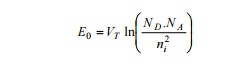Where: E 0 is the zero bias junction voltage, VT the thermal voltage of 26mV at room temperature,

ND  and  NA  are th e impurity concentrations and

ni  is the intrinsic c oncentration.

A suitable positive voltage (forward bias) applied between the two ends of the PN junction can supply the free electrons and holes with the extra en ergy. The external voltage required to overcome this potential barrier that now exists is very much dependent upon the type of semiconduc tor material used and its actual temperature. Typically at room temperature the voltage acro ss the depletion layer for silicon is about 0 .6 - 0.7 volts and for germanium is about 0.3 - 0.35 volts. This potential barrier will always exist even if the device is not connected to an y external power source.

The significance of t his built-in potential across the junction is th at it opposes both the flow of holes and elect rons across the junction and is why it is c alled the potential barrier. In practice, a PN j unction is formed within a single crystal of material rather than just simply joining or fusing together two separate pieces. Electrical contacts are also fused onto either side o f the crystal to enable an electrical connect ion to be made to an external circuit. Then th e resulting device that has been made is cal led a PN junction Diode or Signal Diode.

DEPLETION LAYER PN JUNCTION

If one side of crystal pure semiconductor Si(silicon) or Ge(Germ anium) is doped with acceptor impurity atoms and the other side is doped with donor impurity atoms , a PN junction is formed as shown in figure. P region has high concentration of holes a nd N region contains large number of electrons.Figure1.3 Depletion Layer PN Junction

As soon as the junction is formed, free electrons and holes cross through the junction by the process of diffusion. During this process, the electrons crossing the junction from N-region into P-region , recombine with holes in the P-region very close to the junction. Similarly holes crossing the junction fr om the P-region into the N-region, recombine with electrons in the N- region very close to the junction. Thus a region is formed, whic h does not have any mobile charge very close to th e junction. This region is called the depletion layer of PN junction.

In this region, on th e left side of the junction, the acceptor at oms become negative ions and on the right side of the junction, the donor atoms become positive ions as shown in figure.

FUNCTION OF DEPL ETION LAYER OF PN JUNCTION

An electric field is set up, between the donor and acceptor ions in the depletion layer of the pn junction .The potenti al at the N-side is higher than the potential at P-side. Therefore electrons in the N- side are prevented to go to the lower potential of P -side. Similarly, holes in the P-side find themselves at a lower potential and are prevented to cro ss to the N-side. Thus, there is a barrier at the ju nction which opposes the movement of the majority charge carriers.

The difference of potential from one side of the barrier to the other side of the barrier is called potential barrier. Th e potential barrier is approximately 0.7V for a silicon PN junction and 0.3V for germanium PN junction. The distance from one side of th e barrier to the other side is called the width of the barrier, which depends on the nature of the material.

QUANTITATIVE THEORY OF P-N DIODE CURRENTS

To derive the expressio n for the total current as function of applie d voltage (neglect the barrier width)When diode is fo rward biased, holes injected from the p to n material. The concentration pn of holes in the n-side is increased above equilibrium value pno is  the  diffusion  length  for  holes  in  the  N-material  and  the  injected  or  excess concentration at x=0 isEquation (2) shows the exponential decrease of density pn(x) with distance x into the N-material.From equation 3 hole current decreases exponentially with distance. It is depending on Pn(0) , because it is a function of applied voltage. Ipn depends on applied voltage or injected concentration is a function of voltage.

Law of Junction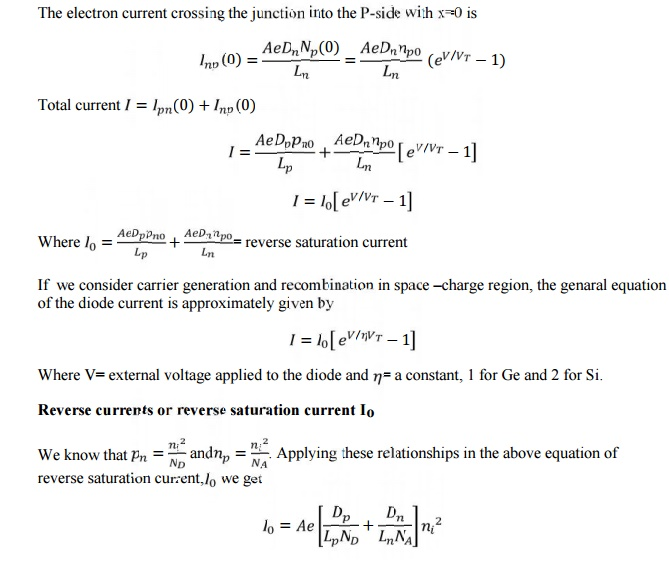1 DIODE CURRENT EQUATION

The diode current equation relating the voltage V and current I is given by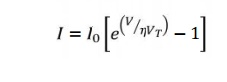where

I – diode current

Io – diode reverse saturation current at room temperature

V – External voltage applied to the diode

η - a constant, 1 for Ge and 2 for Si VT = kT/q = T/11600, thermal voltage

K – Boltzmann‘s constant (1.38066x10^-23 J/K) q – Charge of electron (1.6x10^-19 C)

T – Temperature of the diode junction

At room temperature (T=300 K), VT = 26mV. Substituting this value in current equation,DRIFT AND DIFFUSION CURRENTS

·        The flow of charge (ie) current through a semiconductor material are of two types namely drift & diffusion. (ie) The net current that flows through a (PN junction diode) semiconductor material has two components

(i) Drift current  (ii) Diffusion current

1 DRIFT CURRENT

·   When an electric field is applied across the semiconductor material, the charge carriers attain a certain drift velocity Vd , which is equal to the product of the mobility of the charge carriers and the applied Electric Field intensity E .

Drift velocity Vd = mobility of the charge carriers X Applied Electric field intensity.

·   Holes move towards the negative terminal of the battery and electrons move towards the positive terminal of the battery. This combined effect of movement of the charge carriers constitutes a current known as ― the drift current.

·   Thus the drift current is defined as the flow of electric current due to the motion of the charge carriers under the influence of an external electric field.

·        Drift current due to the charge carriers such as free electrons and holes are the current passing through a square centimeter perpendicular to the direction of flow.Where,        n -  Number of free electrons per cubic centimetre.

P -  Number of holes per cubic centimetre

μ(n) – Mobility of electrons in cm2 / Vs

μ (p) – Mobility of holes in cm2 / Vs

E – Applied Electric filed Intensity in V /cm

q – Charge of an electron  = 1.6 x 10-19 coulomb.

2 DIFFUSION CURRENT

·     It is possible for an electric current to flow in a semiconductor even in the absence of the applied voltage provided a concentration gradient exists in the material.

·     A concentration gradient exists if the number of either elements or holes is greater in one region of a semiconductor as compared to the rest of the Region.

Figure 1.4 (a) Exess hole concentration varying along the axis in an N-type semiconductor bar, (b) The resulting diffusion current

→ In a semiconductor material the change carriers have the tendency to move from the region of higher concentration to that of lower concentration of the same type of charge carriers.

Thus the movement of charge carriers takes place resulting in a current called diffusion current.

As indicated in fig a, the hole concentration p(x) in semiconductor bar varies from a high value to a low value along the x-axis and is constant in the y and z directions.

Diffusion current density due to holes Jp   is given bySince the hole density p(x) decreases with increasing x as shown in fig b, dp/dx  is negative and the        minus sign in equation is needed in order that Jp has positive sign in the positive x direction.

Diffusion current density due to the free electrons is given byDn and Dp – diffusion coefficient for electrons and holes

Total Current

The total current in a semiconductor is the sum of both drift and diffusion currents that is given by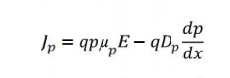Similarly the total current density for an N type semiconductor is given byFORWARD BIAS CONDITION

When positive terminal of the battery is connected to the P-type and negative terminal to N-type of the PN junction diode that is known as forward bias condition.

Operation

The applied potential in external battery acts in opposition to the internal potential barrier which disturbs the equilibrium.

As soon as equilibrium is disturbed by the application of an external voltage, the Fermi level is no longer continuous across the junction.

Under the forward bias condition the applied positive potential repels the holes in P type region so that the holes move towards the junction and the applied positive potential repels the electrons in N type region so that the electrons move towards the junction.

When the applied potential is more than the internal barrier potential the depletion region and internal potential barrier disappear.Figure 1.5 PN junctions under forward bias

V-I Characteristics

As the forward voltage increased for VF < Vo, the forward current IF almost zero because the potential barrier prevents the holes from P region and electrons from N region to flow across the depletion region in opposite direction.Figure 1.6 V-I characteristics of a diode under forward bias

For VF > Vo, the potential barrier at the junction completely disappears and hence, the holes cross the junction from P to N type and electrons cross the junction to opposite direction, resulting large current flow in external circuit.

A feature noted here is the cut in voltage or threshold voltage VF below which the current is very small.At this voltage the potential barrier is overcome and the current through the junction starts to increase rapidly.

Cut in voltage is 0.3V for germanium and 0.7 for silicon.

UNDER REVERSE BIAS CONDITION

When the negative terminal of the battery is connected to the P-type and positive terminal to N-type of the PN junction diode that is known as forward bias condition.

Operation

The holes from the majority carriers of the P side move towards the negative terminal of the battery and electrons which from the majority carrier of the N side are attracted towards the positive terminal of the battery.Figure 1.7 PN junctions under reverse bias

Hence, the width of the depletion region which is depleted of mobile charge carriers increases. Thus, the electric field produced by applied reverse bias, is in the same direction as the electric field of the potential barrier.

Hence the resultant potential barrier is increased which prevents the flow of majority carriers in both directions. The depletion width W is proportional to under reverse bias.

V-I characteristics

Theoretically no current flow in the external circuit. But in practice a very small amount of current of the order of few microamperes flows under reverse bias.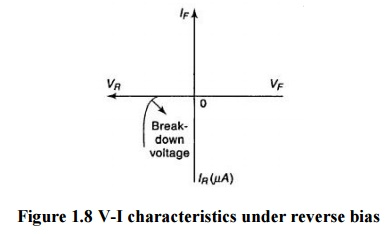Figure 1.8 V-I characteristics under reverse bias

Electrons forming covalent bonds of semiconductor atoms in the P and N type regions may absorb sufficient energy from heat and light to cause breaking covalent bonds. So electron hole pairs continuously produced.

Consequently the minority carriers electrons in the P region and holes in the N region, wander over to the junction and flow towards their majority carrier side giving rise a small reverse current. This current is known as reverse saturation current Io.

The magnitude of this current is depends on the temperature because minority carr ier is thermally broken covalent bonds.

DIODE CHARACTERISTICS

1. Maximum Forward Current

The Maximum Forward Current (IF(max)) is as its name implies the maximum forward current allowed to flow through the device. When the diode is conducting in the forward bias condition, it has a very small "ON" resistance across the PN junction and therefore, power is dissipated across this junction (Ohm´s Law) in the form of heat. Then, exceeding its (IF(max)) value will cause more heat to be generated across the junction and the diode will fail due to thermal overload, usually with destructive consequences. When operating diodes around their maximum current ratings it is always best to provide additional cooling to dissipate the heat produced by the diode.

For example, our small 1N4148 signal diode has a maximum current rating of about 150mA with a power dissipation of 500mW at 25oC. Then a resistor must be used in series with the diode to limit the forward current, (IF(max)) through it to below this value.

2. Peak Inverse Voltage

The Peak Inverse Voltage (PIV) or Maximum Reverse Voltage (VR(max)), is the maximum allowable Reverse operating voltage that can be applied across the diode without reverse breakdown and damage occurring to the device. This rating therefore, is usually less than the "avalanche breakdown" level on the reverse bias characteristic curve. Typical values of VR(max) range from a few volts to thousands of volts and must be considered when replacing a diode.The peak inverse voltage is an important parameter and is mainly used for rectifying diodes in AC rectifier circuits with reference to the amplitude of the voltage were the sinusoidal waveform changes from a positive to a negative value on each and every cycle.

3. Forward Power Dissipation

Signal diodes have a Forward Power Dissipation, (PD(max)) rating. This rating is the maximum possible power dissipation of the diode when it is forward biased (conducting). When current flows through the signal diode the biasing of the PN junction is not perfect and offers some resistance to the flow of current resulting in power being dissipated (lost) in the diode in the form of heat. As small signal diodes are nonlinear devices the resistance of the PN junction is not constant, it is a dynamic property then we cannot use Ohms Law to define the power in terms of current and resistance or voltage and resistance as we can for resistors. Then to find the power that will be dissipated by the diode we must multiply the voltage drop across it times the current flowing through it: PD = VxI

4. Maximum Operating Temperature

The Maximum Operating Temperature actually relates to the Junction Temperature (TJ) of the diode and is related to maximum power dissipation. It is the maximum temperature allowable before the structure of the diode deteriorates and is expressed in units of degrees centigrade per Watt, ( oC/W ). This value is linked closely to the maximum forward current of the device so that at this value the temperature of the junction is not exceeded. However, the maximum forward current will also depend upon the ambient temperature in which the device is operating so the maximum forward current is usually quoted for two or more ambient temperature values such as 25oC or 70oC.

SWITCHING CHARACTERISTICS

Diodes are often used in switching mode. When the applied bias voltage to the PN diode is suddenly reversed in opposite direction and it reaches a steady state at a interval of time that is called the recovery time.

Forward recovery time is defined is the time required the forward voltage or current to reach a specified value after switching diode from its reverse to forward biased state.

When PN diode is forward biased the minority electrons concentration in P region is linear. If the junction is suddenly reversed at t1 then because of stored electronic charge, the reverse current IR is initially of the same magnitude as forward current IF.

The diode will continue to conduct until the injected or excess minority carrier density (p-po) or (n-no) has dropped to zero shown in fig. c.

In fig. b the applied voltage Vi = VF for the time up to t1 is in the direction to forward bias the diode. The resistance RL is large so that the drop across RL is large when compared to the drop across diode. Then the current is I= VF / RL = IF.

At time t=t1 the input voltage is reversed to the value of –VR current does not become zero and the value is I= VR / RL = IR shown in fig d..

During the time interval from t1 to t2 the injected minority carriers have remained stored and hence this interval is called the storage time (t1).

After the instant t=t2, the diode gradually recovers and ultimately reaches the steady state. The time interval between t2 and instant t3 when the diode has recovered nominally is called the transition time tt.

The recovery said to have completed (i) when even the minority carriers remote from the junction have difference to the junction and crossed it. (ii) when the junction transition capacitance C across the reverse biased junction has got charged through the external resistor RL to the voltage –VR.

For commercial switching type diodes the reverse recovery time trr ranges from less than 1ns up to as high as 1us. In order to minimize the effect of reverse current the time period of the operating frequency should be a minimum of approximately 10 times trr.Figure 1.9 b) Switching characteristics of PN diode

For example if diode has trr of 2ns its operating frequency isThe reverse recovery time can be reduced b shortening the length of the P region in a PN junction diode.

The stored storage and switching time can be reduced by introduction of gold impurities into junction diode by diffusion. The gold doping also called a life time killer, increases the recombination rate and removes the stored minority carriers.This technique is used to produce diodes and other active devices for high speed applications.

APPLICATION OF PN DIODE

·        Can be used as rectifier in DC Power Supplies.

·        In Demodulation or Detector Circuits.

·        In clamping networks used as DC Restorers

·        In clipping circuits used for waveform generation.

·        As switches in digital logic circuits.

·        In demodulation circuits.

Study Material, Lecturing Notes, Assignment, Reference, Wiki description explanation, brief detail
Electronic Devices : Semiconductor Diode : Semiconductor Diode |

Related Topics